## GPU Gems 2

GPU Gems 2 is now available, right here, online. You can purchase a beautifully printed version of this book, and others in the series, at a 30% discount courtesy of InformIT and Addison-Wesley.

The CD content, including demos and content, is available on the web and for download.

# Chapter 47. Flow Simulation with Complex Boundaries

Wei Li
Siemens Corporate Research

Zhe Fan
Stony Brook University

Xiaoming Wei
Stony Brook University

Arie Kaufman
Stony Brook University

## 47.1 Introduction

Physically based fluid flow simulation is commonplace in many prediction and scientific computation problems. It also greatly improves the visual fidelity in computer graphics applications. However, an accurate simulation is computationally expensive. In this chapter, we present a physically plausible yet fast fluid flow simulation approach based on the Lattice Boltzmann Method (LBM) (Chen and Doolean 1998). Figure 47-1 shows the results of fluid simulation around different obstacles. In Figure 47-1a, the obstacle is a static vase, while in Figure 47-1b, it is a sphere that moves toward the top right. Figure 47-1c and Figure 47-1d are two snapshots showing a jellyfish swimming from right to left. Note that when the jellyfish deforms its body, the liquid-solid boundary also deforms. To visualize the flow field, we inject a slice of colored particles and advect them according to the velocity field of the flow. All the computations, the simulation, the generation of the boundaries, the advection, and the rendering of the particles are executed on the GPU in real time.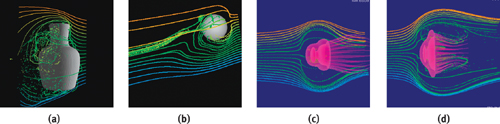Figure 47-1 Flow Fields Simulated on the GPU Using Different Obstacles

The LBM model is composed of many nodes arranged on Cartesian grids, usually called a lattice. Each node is associated with several attributes. At discrete time steps, the attributes are updated to determine the dynamics of a flow field. The basic computation of the LBM naturally fits the stream-processing framework of the GPU, hence it is not very difficult to express the LBM equations using GPU programs. However, a straightforward translation results in a very slow implementation. In this chapter, we present various algorithm-level and machine-level optimizations. With the proper optimizations, a GPU-based flow simulation can be an order of magnitude faster than its CPU counterpart.

One of the advantages of the LBM is that it is relatively easy to handle complex, moving, and deformable boundaries. However, the boundaries must be voxelized to discrete boundary nodes that are aligned with the LBM lattice. Voxelization of moving and deforming boundaries primarily involves finding intersections of the boundary polygons with the LBM lattice. Voxelization must be repeated whenever the boundaries change. If CPU-based voxelization is used with GPU-based LBM, the transfer of boundary nodes from main memory to graphics memory becomes a bottleneck. To address these problems, we propose a fast voxelization algorithm on the GPU and use it to model the interaction of complex obstacles and even living objects with the flow.

## 47.2 The Lattice Boltzmann Method

Figure 47-2 shows a 2D model with the nodes represented as green dots. The figure shows only 12 nodes, but in practice, we need thousands or even millions of nodes to generate a delicate flow field. Each node is connected to its direct neighbors with vectors, denoted as e qi and shown as black arrows in Figure 47-2. There is also an e qi pointing to the node itself (not shown in the figure). The model in Figure 47-2 is called D2Q9, because each node has 9 e qi vectors. Each node is associated with various attributes, such as packet distribution fqi , density , and velocity u. We don't need to worry about their physical meanings. All we need to know is that densities and velocities are derived from packet distributions, and velocities are the output that we need for our application. Interested readers may find more details about LBM in the literature, such as Chen and Doolean 1998 and Wei et al. 2004. For each node, there is one packet distribution for every e qi vector. Therefore in this 2D model, each node has 9 packet distributions.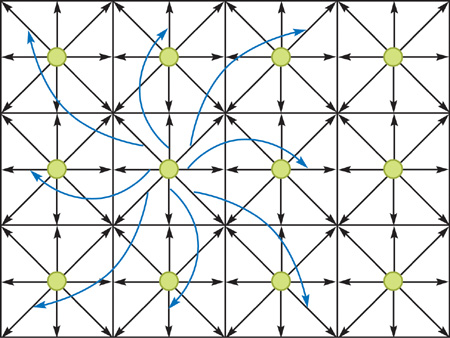Figure 47-2 A 2D LBM Model

We are most interested in 3D flow. The 3D model that we use in this chapter is called D3Q19 because it has 19 packet distributions for each node. These packet distributions account for most of the memory requirements of the LBM model. All the attributes are updated at every time step, using the values of the previous step. Every packet distribution moves along its e qi vector to the neighbor node and replaces the packet distribution of the neighbor node, except the one pointing to the node itself, as indicated by the blue arrows in Figure 47-2. This is called propagation or streaming.

## 47.3 GPU-Based LBM

#### 47.3.1 Algorithm Overview

To compute the LBM equations on graphics hardware, we divide the LBM lattice and group the packet distributions fqi into arrays according to their velocity vectors e qi . All packet distributions of the same velocity vector are grouped into an array, maintaining the layout of the original lattice. Figure 47-3 shows the division of a 2D model into a collection of arrays (one per velocity vector e qi ). The division of a 3D model is similar. We store the arrays as 2D textures. For a 2D model, all such arrays are naturally 2D; for a 3D model, each array forms a volume. The volume is treated as a set of slices and is stored as a stack of 2D textures or a large tiled 2D texture. All the other variables are stored similarly in 2D textures. To update these values at each time step, we render quadrilaterals mapped with those textures, so that a fragment program running at each texel computes the LBM equations.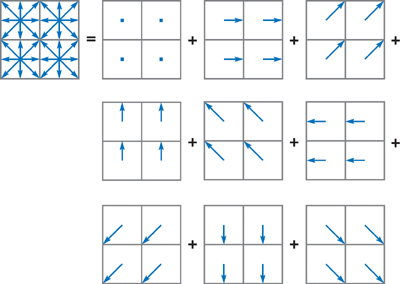Figure 47-3 Division of a D2Q9 Model According to Its Velocity Directions

Figure 47-4 is a diagram of the data flow of the LBM computation on the GPU; green boxes represent the textures storing lattice properties, and blue boxes represent the operations. An LBM update iteration starts with the packet distribution textures as inputs. Density and velocity textures are then dynamically generated from the distribution textures. Next, intermediate distributions, called equilibrium distributions, are obtained from the densities and velocities. New distributions are then computed from the input distributions and the equilibrium distributions according to the collision and the streaming equations. Finally, the boundary and outflow conditions are used to update the distribution textures. The updated distribution textures are then used as inputs for the next simulation step. Readers can find the details of these computations in Wei et al. 2004.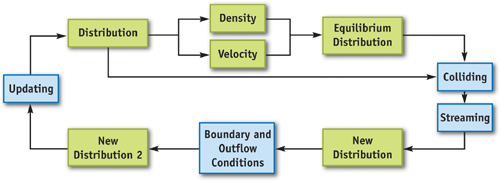Figure 47-4 Flow Chart of LBM Computation on the GPU

#### 47.3.2 Packing

During the simulation, textures are updated dynamically at every step by copying from or binding to the frame buffer (or an off-screen buffer). In current graphics hardware, it is most efficient to use 8-bit-per-component RGBA (or BGRA) textures. Each RGBA texel has four channels, so it can store up to four scalars or a vector with up to four components.

We pack multiple variables into each texel. For example, four packet distributions, fqi , from different directions are stored in a single RGBA texel. It is important to pack those variables involved in the same LBM equations into the same texture or even the same texel, if possible, in order to reduce the number of texture fetches. This also improves data locality and texture-cache coherence.

Table 47-1 lists the contents of the textures packed with the variables of the D3Q19 model, including densities, velocities, and packet distributions. In texture u , vx , vy , and vz are the three components of the velocity stored in the RGB channels, while the density is stored in the alpha channel. The rows in Table 47-1 for textures f 0 through f 4 show the packing patterns of the packet distributions fqi . The distribution in the direction of (x, y, z) is f (x,y,z). Note that we pack pairs of distributions of opposite directions into the same texture. There are two reasons for this. First, when we handle complex or moving boundaries, neighboring distributions at opposite directions are needed to evaluate the effects on a boundary link. Second, when programming the fragment processor, we typically need to pass the corresponding velocity vector e qi as an argument of the fragment program. When opposite distributions are packed together, just two e qi 's are needed, instead of four, and the other two are easily inferred.

#### Table 47-1. Packed LBM Variables of the D3Q19 Model

 Texture R G B A u vx vy vz f 0 f (1, 0, 0) f (–1, 0, 0) f (0, 1, 0) f (0, –1, 0) f 1 f (1, 1, 0) f (–1, –1, 0) f (1, –1, 0) f (–1, 1, 0) f 2 f (1, 0, 1) f (–1, 0, –1) f (1, 0, –1) f (–1, 0, 1) f 3 f (0, 1, 1) f (0, –1, –1) f (0, 1, –1) f (0, –1, 1) f 4 f (0, 0, 1) f (0, 0, –1) f (0, 0, 0) unused

Instead of using a stack of 2D textures to represent a volume, we tile the slices into a large 2D texture that can be considered a "flat" volume. Similar approaches have been reported in the literature, for example, in Harris et al. 2003. One advantage of the flat volume is the reduced amount of texture switching. Using the flat volume is critical in two stages of our algorithms: particle advection and boundary node generation. The conversion from the volume coordinates (x, y, z) to the coordinates (u, v) of the flattened texture is as follows:

Equation 1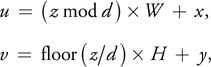where W and H are dimensions of each slice in the volume, and every set of d slices is tiled in a row along the x direction in the flat volume. The computation of Equation 1 can be done either in the fragment program or via a 1D texture lookup.

#### 47.3.3 Streaming

Recall that each packet distribution with nonzero velocity propagates to a neighbor node at every time step. On modern GPUs, the texture unit can fetch texels at arbitrary positions indicated by texture coordinates computed by the fragment program. If a distribution fqi is propagated along vector e qi , we simply fetch from the distribution texture at the position of current node minus e qi . Because the four channels are packed with four distributions with different velocity vectors, four fetches are needed for each fragment.

Figure 47-5 shows an example of the propagation of distribution texture f 1, which is packed with f (1, 1, 0), f (–1, –1, 0), f (1, –1, 0), and f (–1, 1, 0). The fragment program fetches texels by adding the negative values of the velocity directions to the texture coordinates of the current fragment and extracting the proper color component.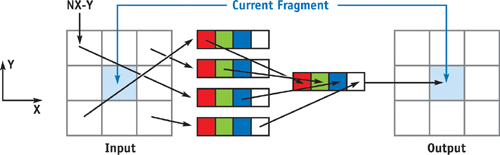Figure 47-5 Streaming of Packet Distribution

To propagate using the flat volume, for each channel we can add the 3D position of the fragment to the negative of the corresponding e qi , then convert it to the texture coordinate of the flat volume according to Equation 1 before fetching. However, this requires execution of Equation 1 four times per fragment. We can push the coordinates' conversion to the vertex level, because for each channel inside a slice, the velocity vectors are the same. We can either assign each vertex of the proxy quad four texture coordinates containing the converted values or generate the texture coordinates with a vertex program.

## 47.4 GPU-Based Boundary Handling

The previous computations apply only to internal nodes, away from any obstacle boundaries. To make the simulation work properly, and to make the flow interact with obstacles, we need to consider boundary conditions. To handle an obstacle boundary, we need to compute the intersections of the boundary surface with all the LBM lattice links. For static obstacles, the intersections can be precomputed, whereas for moving or deformable boundaries, the intersection positions change dynamically. The boundary description can be either continuous, such as a polygonal mesh or a higher-order surface, or discrete, such as a voxel volume. Regardless, the handling of the boundary conditions requires discrete boundary nodes to be aligned with the LBM lattice. Even if the boundary representation is already volumetric, it has to be revoxelized whenever it moves or deforms. One solution is to compute the intersection and voxelization on the CPU and then transfer the computed volumetric boundary information from the main memory to the graphics memory. Unfortunately, both the computation and the data transfer are too time-consuming for interactive applications.

In the following sections, we first propose a general-purpose GPU-based voxelization algorithm that converts an arbitrary model into a Cartesian grid volume. Then we discuss the handling of three different boundary conditions, while focusing on arbitrary complex boundaries that can move and deform. The generation of the boundary nodes of arbitrary boundaries is performed by extending our general-purpose GPU-based voxelization.

#### 47.4.1 GPU-Based Voxelization

An intuitive voxelization approach is the slicing method, which sets the near and far clip planes so that for each rendering pass, only the geometry falling into the slab between the two clip planes is rendered (Fang and Chen 2000). This creates one slice of the resulting volume. The clip planes are shifted to generate subsequent slices. Obviously, for this slicing method, the number of rendering passes is the same as the number of slices in the volume. In most cases, the boundaries are sparse in a volume. In other words, only a small percentage of voxels are intersected by the boundary surfaces. There is no need to voxelize the "empty" space that corresponds to nonboundary voxels.

Our GPU-based voxelization avoids this slicing. Instead, we adapt the idea of depth peeling (Everitt 2001) used for order-independent transparency, in which the depth layers in the scene are stripped away with successive rendering passes. In the first pass, the scene is rendered normally, and we obtain the layer of nearest fragments—or equivalently, voxels. From the second rendering pass, each fragment is compared with a depth texture obtained from the depth buffer of the previous pass. A fragment reaches the frame buffer only if its depth value is greater than that of the corresponding pixel in the depth texture, while the ordinary depth test is still enabled. Therefore, the second pass generates the second-nearest layer, and so on. The process continues until no fragment is farther away than the corresponding pixel in the depth texture. This condition is best determined by using a hardware occlusion query, which returns the number of pixels written to the frame buffer. The number of rendering passes of the depth-peeling algorithm is the number of layers plus one, which typically is significantly smaller than the number of slices.

When rendering order-independent transparent objects, all the layer images are blended in depth order. In contrast, for voxelization, we want the layers to be separated, which is similar to a layered depth image (Shade et al. 1998). The pixels of the layered images are the attributes of the corresponding voxels. The first attribute is the 3D position, and the other attributes depend on the application. Assume that the maximum size along any of the major axes of the object being voxelized is D. We allocate an off-screen buffer with width and height equal to the maximum number of layers times D and the number of attributes times D, respectively. Then, between rendering passes, we translate the viewport so that the layer images do not overlap but are tiled as tightly as possible. We apply the peeling process three times; each time, the image plane is orthogonal to one of the major axes. That is, we perform the peeling from three orthogonal views to avoid missing voxels. As a result, some of the voxels may be rendered more than once. However, the replication does not affect the accuracy. The images containing the voxel attributes are then copied to a vertex array allocated in video memory (using OpenGL extensions such as ARB_pixel_buffer_object and ARB_vertex_buffer_object [NVIDIA 2004]). Note that different types of voxel attributes are copied to different locations inside the vertex array. We may avoid the copying if either render-to-vertex-array or vertex texture fetch is available. Figure 47-6 illustrates the process in 2D. The line segments are the boundary lines (surfaces in 3D). The small red, green, and blue boxes represent the voxels generated when the boundary lines are projected onto the layered off-screen buffers. Note that the red boundary will only result in two voxels if it is only rendered into layer X.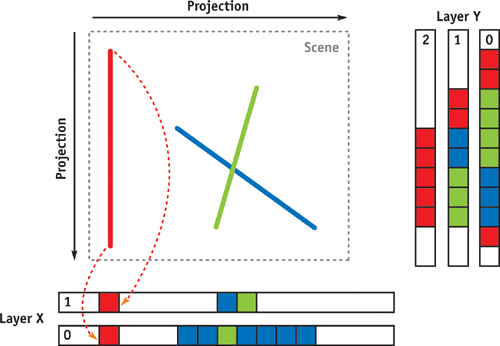Figure 47-6 Voxelization Based on Depth Peeling

The vertex array is essentially an array of voxel positions and other attributes, which can generate all the boundary voxels for further processing. We use the vertex array because current GPU fragment programs cannot scatter (that is, the output location of a fragment is fixed). Therefore, we must rely on the transformation capability of vertex programs. We convert the vertex array into a flat volume by rendering each vertex as a point of size 1, so that each voxel has a footprint of 1 pixel. All the vertices of the array pass through a vertex program that translates each voxel properly according to its z coordinate, using equations similar to Equation 1. The frame buffers for the depth peeling are initialized with large numbers. If a pixel is not covered by any boundary voxels, then the corresponding vertex created from the pixel falls far away from the view frustum and is clipped.

#### 47.4.2 Periodic Boundaries

For periodic boundaries, outgoing packet distributions wrap around and reenter the lattice from the opposite side. In practice, periodic boundaries are actually computed during the propagation of the packet distributions. If a periodic boundary face is orthogonal to the x or y axis, we call it an in-slice periodic boundary face, because a distribution on the face is copied to the opposite side of the lattice but stays inside the same xy slice. For in-slice periodic boundaries, we simply apply a modulo operation to the texture coordinates by the width or the height of each slice. If a periodic boundary face is perpendicular to the z axis, we call it an out-slice periodic boundary face, for which we need to copy distribution textures of one slice to another.

A naive implementation of the in-slice periodic boundary condition is to apply the modulo operation to the texture coordinates of all the distribution texels. However, this can be very slow. Therefore, one optimization we use first propagates without the periodic boundary condition. Then we draw strips of single-texel width that only cover the boundary and apply the modulo operation. (See Chapter 34 of this book, "GPU Flow-Control Idioms," for more information on this technique.) In this way, the cost for computing is negligible, because the periodic boundary nodes account for only a small percentage of the lattice.

#### 47.4.3 Outflow Boundaries

For outflow conditions, some packet distributions of the boundary nodes propagate outside of the lattice and can simply be discarded. However, these nodes also expect some packet distributions to propagate inward from fictitious nodes just outside the boundary. One solution is to copy the distribution of the internal nodes to those boundary nodes, according to Equations 2, 3, and 4, in which f(B)(i, j, k) is the distribution of a boundary node in the velocity direction of (i, j, k); f(I)(i, j, k) is the distribution of the corresponding internal node; and i, j, k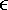{-1, 0, 1}. For example, boundary nodes on slice 0 are copied from internal nodes two slices away, which are on slice 2.

For an outflow face orthogonal to the x axis:

Equation 2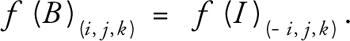For an outflow face orthogonal to the y axis:

Equation 3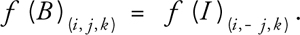For an outflow face orthogonal to the z axis:

Equation 4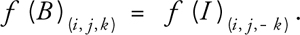Similar to periodic boundaries, an outflow boundary condition is applied by drawing single-texel-wide stripes. Note that when packing the distributions, we guarantee that f (i, j, k) and f (–i, j, k) of the same node are in the same texture, as well as the pairs f (i, j, k) and f (i, –j, k), f (i, j, k) and f (i, j, –k). You can easily verify these with Table 47-1. Therefore, each boundary distribution texture copies from only one distribution texture. To flip the distributions around the major axes, we use the swizzling operator to rearrange the order of the color elements.

#### 47.4.4 Obstacle Boundaries

For obstacle boundaries, we adopt the improved bounce-back rule of Mei et al. 2000, which can handle deformable and moving boundaries. As indicated in Figure 47-7, all packet distributions with corresponding links that are intersected by a boundary are updated using the curved boundary equations rather than the standard LBM equations. For example, the link between the two nodes in Figure 47-7 is intersected by a boundary, hence the boundary rules are applied to the packet distributions marked in blue, f 1 and f 2. Again, we don't need to worry about the details of these equations; interested readers will find these equations in Mei et al. 2000. We just need to know that the boundary equations require two values in addition to the values of f 1 and f 2 at the previous time step. The first value is the exact intersection location, which is described by the ratio , where = t 1/(t 1 + t 2) for f 1, and = t 2/(t 1 + t 2) for f 2. The second value is the moving speed at the intersection, u w , which is useful for moving and deformable boundaries. Note that for each boundary link, the boundary condition affects two distributions, one on each side of the boundary. The two distributions are in opposite directions, but they are colinear with the link. We refer to them as boundary distributions.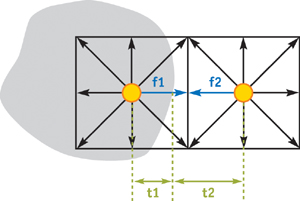Figure 47-7 Curved-Wall Boundary in LBM Lattice

To generate the boundary information, we first create a voxelization of the boundaries using the method described in the previous section. Besides the position of the voxels, we also need the wall velocity u w , as well as the coefficients of polygon plane equations, which will be used to compute . That is, we need three attributes in total. To preserve accuracy, we treat these attributes as texture coordinates when rendering the vertex array in the next step.

In practice, we don't explicitly generate the flat volume of the boundary voxels. Rather, we combine the generation with the computation of the boundary conditions, by rendering the boundary vertex array directly into the off-screen buffer containing the propagated new distributions and then applying the fragment program for complex boundary conditions. Note that in most cases only one node of each boundary link is covered by a voxel from the generated voxel array. However, we need each boundary node to receive a fragment so that the boundary distributions are updated. Therefore, for each packed distribution texture, we render the voxel array three times. In the first pass, they are rendered normally, covering those voxels in the generated voxel array. In the second pass, we first set the color mask so that only the R and G channels can be modified. Then we apply a translation to all the voxels using a vertex program. The translated offset is computed according to the following rule: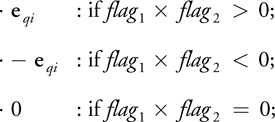where flag 1 = (posx , posy , posz , 1) · (A, B, C, D), flag 2 = (A, B, C) · e qi , and · represents a dot product. The coordinates (posx , posy , posz ) represent the 3D position of the voxel without translation. The boundary surface inside the voxel is defined by Ax + By + Cz + D = 0, where (A, B, C) is a normalized plane normal pointing from the solid region to the liquid region. The value e qi is the velocity vector associated with the distribution in the red channel. The third pass is similar to the second pass, except that this time the blue and alpha channels are modified, and e qi is the velocity vector corresponding to the blue channel distribution.

In this way, all the boundary nodes are covered by the voxels. We then compute at the beginning of the boundary condition fragment program with the following equations: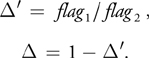The meanings of flag 1 and flag 2 are the same as before. Note that each channel computes its flag 1, flag 2, and independently, with its own e qi . A distribution is a boundary distribution only if, for the corresponding color channel, 1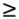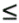0. If it is not a boundary distribution, the fragment program prevents modifying the corresponding color channel by assigning it the old value.

## 47.5 Visualization

To visualize the simulation, we inject particles into the flow field. The positions of the particles are stored in a texture and are updated every time step by a fragment program using the current velocity field. Similar to the generation of boundary voxels, the updated particle positions are then copied to a vertex array residing in the graphics memory for rendering. The whole simulation and rendering cycle is inside the GPU, hence there is no need to transfer large chunks of data between the main memory and the graphics memory. To better display the flow field, we arrange particles in a regular grid before injection and color them according to the position where they enter the flow field, as shown in Figure 47-1. Due to the requirements of the vertex array, the total number of particles is constant during the simulation. We use a fragment program to recycle particles that flow out of the border of the field or stay in a zero-velocity region and place them back at the inlet. If two particles coincide at exactly the same location at the same time, they will never separate during the advection. Visually, we will see fewer and fewer particles. To avoid this, we add a random offset to each particle when placing it at the inlet.

In 2D LBM, there is only one velocity slice, while in 3D LBM, the velocities form a volume. The advection fragment program fetches the velocity indicated by the current position of the particles. Therefore, the fragment program needs to access either a 3D texture or a 2D texture storing the flat volume. We chose the flat volume storage, which is much faster. Please note that if we stored the velocity as a stack of separate 2D textures, the advection would be very difficult.

## 47.6 Experimental Results

We have experimented with our GPU-based LBM implemented using OpenGL and Cg on an NVIDIA GeForce FX 5900 Ultra. This GPU has 256 MB of 425 MHz DDR SDRAM, and its core speed is 400 MHz. The host computer has a Pentium 4 2.53 GHz CPU with 1 GB PC800 RDRAM. All the results related to the GPU are based on 32-bit single-precision computation of the fragment pipeline. For comparison, we have also implemented a software version of the LBM simulation using single-precision floating point, and we measured its performance on the same machine.

Figure 47-8 shows a 2D flow field simulation based on the D2Q9 model with a lattice size of 2562. We insert two circles (shown in red) into the field as the obstacles' boundary conditions. Vortices are generated because of the obstacles. The figure shows only a snapshot of the flow; the flow is relatively stable, but not constant. Figure 47-1 shows 3D flow simulations using the D3Q19 model with a lattice size of 503. Note that our simulation can handle arbitrarily complex and dynamic boundaries, which usually results in a complex flow field. We show only particles injected from a slit, to reduce clutter. The motion of the particles and the ability to change the viewing angle help in understanding the shape of the flow.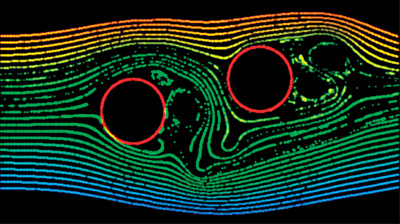Figure 47-8 Particles Advected in a 2D Flow Field Based on the D2Q9 LBM Model

Figure 47-9 shows the time in milliseconds per step of the 3D LBM model as a function of the lattice size, running on both the CPU and the GPU. Note that both the x and y axes are in logarithmic scale. Figure 47-10 compares the two from a different perspective by showing the speedup factor. The time includes both simulation and visualization. Note that there is no need to transfer the velocity field or the particle positions between the main memory and the graphics memory. The time spent on advecting and rendering the particles is negligible with respect to the simulation. The speedup factor varies between 8 and 9 for most of the lattice sizes. When the lattice size approaches 1283, the speedup is as high as 15. We are sure to get higher speedups with more recent GPUs, such as a GeForce 6800-class GPU. The step in the GPU timing curve—as well as in the GPU versus CPU speedup—is good evidence showing their different cache behavior. When the grid size gets bigger and surpasses a certain threshold, cache misses significantly slow down the CPU version. Due to the mostly sequential access patterns of the LBM algorithm and the GPU's optimization for sequential access, the cache-miss rate on the GPU is relatively independent of the grid size, and we don't see such a performance jump. The performance of the CPU version actually drops dramatically for lattice size of exactly power of two, possibly caused by 64K aliasing (Intel 2004). Therefore, we actually use lattices of size power-of-two-plus-one for the measurement. The CPU-based LBM implementation does not utilize SSE.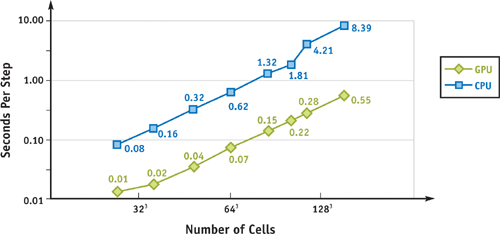Figure 47-9 Time per Step of a D3Q19 LBM Simulation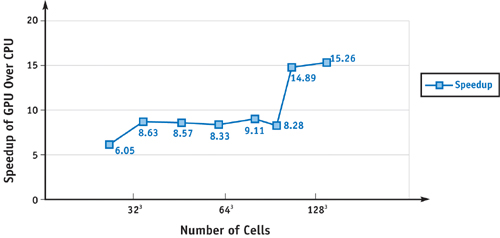Figure 47-10 Speedup of the LBM on the GPU

## 47.7 Conclusion

We have presented a fluid flow simulation using the physically based Lattice Boltzmann Method, accelerated by programmable graphics hardware. The LBM simulation and its boundary conditions are of second-order accuracy in both time and space. Our GPU-based simulation using floating-point computation achieves the same accuracy as the CPU-based LBM simulation, yet it is much faster. Our experimental results have shown that the GPU version is significantly faster (for example, 8 to 15 times faster, for the D3Q19 model) for most lattice sizes in both 2D and 3D simulations. To attain these speeds, we have incorporated several optimization techniques into the GPU method. We have also proposed a GPU-based general-purpose voxelization algorithm and its extension to handle arbitrarily complex and dynamic boundary conditions in real time.

## 47.8 References

Other graphics researchers have explored the approach of solving the Navier-Stokes (NS) equations to simulate amorphous phenomena, such as gases and fluid: Foster and Metaxas 1997, Stam 1999, and Fedkiw et al. 2001. Readers may find a comparison of direct NS solvers with the LBM in Wei et al. 2004. Researchers have also developed GPU-accelerated conjugate gradient solvers that can be used for NS equations: Bolz et al. 2003, Krüger and Westermann 2003. There are also other works on using GPUs to compute fluid dynamics: Harris et al. 2003, Goodnight et al. 2003, and Harris 2004. More details of the GPU-accelerated LBM can be found in Li 2004.

Bolz, J., I. Farmer, E. Grinspun, and P. Schröder. 2003. "Sparse Matrix Solvers on the GPU: Conjugate Gradients and Multigrid." ACM Transactions on Graphics (Proceedings of SIGGRAPH 2003) 22(3), pp. 917–924.

Chen, S., and G. D. Doolean. 1998. "Lattice Boltzmann Method for Fluid Flows." Annual Review of Fluid Mechanics 30, pp. 329–364.

Everitt, C. 2001. "Interactive Order-Independent Transparency." NVIDIA technical report. Available online at http://developer.nvidia.com/object/Interactive_Order_Transparency.html

Fang, S., and H. Chen. 2000. "Hardware Accelerated Voxelization." Computers and Graphics 24(3), pp. 433–442.

Fedkiw, R., J. Stam, and H. W. Jensen. 2001. "Visual Simulation of Smoke." In Proceedings of SIGGRAPH 2001, pp. 15–22.

Foster, N., and D. Metaxas. 1997. "Modeling the Motion of a Hot, Turbulent Gas." In Proceedings of SIGGRAPH 97, pp. 181–188.

Goodnight, N., C. Woolley, G. Lewin, D. Luebke, and G. Humphreys. 2003. "A Multigrid Solver for Boundary Value Problems Using Programmable Graphics Hardware." In Proceedings of the SIGGRAPH /Eurographics Workshop on Graphics Hardware 2003, pp. 102–111.

Harris, M. 2004. "Fast Fluid Dynamics Simulation on the GPU." In GPU Gems, edited by Randima Fernando, pp. 637–665. Addison-Wesley.

Harris, M., W. V. Baxter, T. Scheuermann, and A. Lastra. 2003. "Simulation of Cloud Dynamics on Graphics Hardware." In Proceedings of the SIGGRAPH/Eurographics Workshop on Graphics Hardware 2003, pp. 92–101.

Intel. 2004. "Capacity Limits and Aliasing in Caches." In Chapter 2 of IA-32 Intel Architecture Optimization Reference Manual, pp. 41–43. Available online at http://www.intel.com/design/pentium4/manuals/index_new.htm

Krüger, J., and R. Westermann. 2003. "Linear Algebra Operators for GPU Implementation of Numerical Algorithms." ACM Transactions on Graphics (Proceedings of SIGGRAPH 2003) 22(3), pp. 908–916.

Li, W. 2004. "Accelerating Simulation and Visualization on Graphics Hardware." Ph.D. dissertation, Computer Science Department, Stony Brook University.

Mei, R., W. Shyy, D. Yu, and L.-S. Luo. 2000. "Lattice Boltzmann Method for 3-D Flows with Curved Boundary." Journal of Computational Physics 161, pp. 680–699.

NVIDIA Corporation. 2004. OpenGL Extension Specifications. Available online at http://developer.nvidia.com/object/nvidia_opengl_specs.html

Shade, J., S. J. Gortler, L. He, and R. Szelisk. 1998. "Layered Depth Images." In Proceedings of SIGGRAPH 98, pp. 231–242.

Stam, J. 1999. "Stable Fluids." In Proceedings of SIGGRAPH 99, pp. 121–128.

Wei, X., W. Li, K. Mueller, and A. Kaufman. 2004. "The Lattice-Boltzmann Method for Gaseous Phenomena." IEEE Transactions on Visualization and Computer Graphics 10(2), pp. 164–176.

Many of the designations used by manufacturers and sellers to distinguish their products are claimed as trademarks. Where those designations appear in this book, and Addison-Wesley was aware of a trademark claim, the designations have been printed with initial capital letters or in all capitals.

The authors and publisher have taken care in the preparation of this book, but make no expressed or implied warranty of any kind and assume no responsibility for errors or omissions. No liability is assumed for incidental or consequential damages in connection with or arising out of the use of the information or programs contained herein.

NVIDIA makes no warranty or representation that the techniques described herein are free from any Intellectual Property claims. The reader assumes all risk of any such claims based on his or her use of these techniques.

The publisher offers excellent discounts on this book when ordered in quantity for bulk purchases or special sales, which may include electronic versions and/or custom covers and content particular to your business, training goals, marketing focus, and branding interests. For more information, please contact:

U.S. Corporate and Government Sales
(800) 382-3419
corpsales@pearsontechgroup.com

International Sales
international@pearsoned.com

Visit Addison-Wesley on the Web: www.awprofessional.com

GPU gems 2 : programming techniques for high-performance graphics and general-purpose
computation / edited by Matt Pharr ; Randima Fernando, series editor.
p. cm.
Includes bibliographical references and index.
ISBN 0-321-33559-7 (hardcover : alk. paper)
1. Computer graphics. 2. Real-time programming. I. Pharr, Matt. II. Fernando, Randima.

T385.G688 2005
006.66—dc22
2004030181

Nalu, Timbury, and Clear Sailing images © 2004 NVIDIA Corporation.

mental images and mental ray are trademarks or registered trademarks of mental images, GmbH.

All rights reserved. No part of this publication may be reproduced, stored in a retrieval system, or transmitted, in any form, or by any means, electronic, mechanical, photocopying, recording, or otherwise, without the prior consent of the publisher. Printed in the United States of America. Published simultaneously in Canada.

For information on obtaining permission for use of material from this work, please submit a written request to:

Pearson Education, Inc.
Rights and Contracts Department
One Lake Street